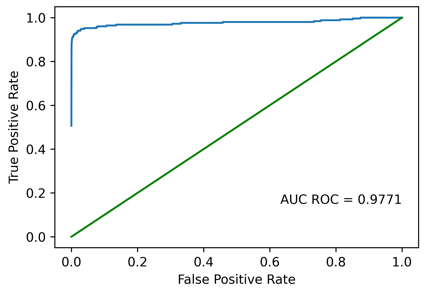# Plot line is cut off

Hello,

I am trying to create some nice AUCROC plots using matplotlib. When I plot the graphs, they seem somehow cut off at the left side where they should go to (0,0) where the green line starts as well. I appended a 0 in front of the x- and y-values to make sure that (0,0) is part of the plot.
I tried shifting the axes’ limits to the left, but I am out of ideas.

Thanks for any ideasThe graph shows (0, 0). If you are referring to the blue line, Matplotlib just plots the data that you’ve given it, so if you don’t see (0, 0), you should double-check the input to the plot. Without any code, there’s not much else to suggest.

I found the problem when I tried to set up a minimum working example. The problem was that I appended a 0 to all lists, regardless of whether they already go through (0,0) or not. For lists like

``````x = [0.1, 0.5, 1.0]
y = [0.5, 0.9, 1.0]
``````

this was ok, as they became

``````x = [0.0, 0.1, 0.5, 1.0]
y = [0.0, 0.5, 0.9, 1.0]
``````

and could be plotted as expected. However, when the list already had 0.0 as first x-value like

``````x = [0.0, 0.5, 1.0]
y = [0.5, 0.9, 1.0]
``````

``````x = [0.0, 0.0, 0.5, 1.0]
``````x = [0.0, 1e-10, 0.5, 1.0]